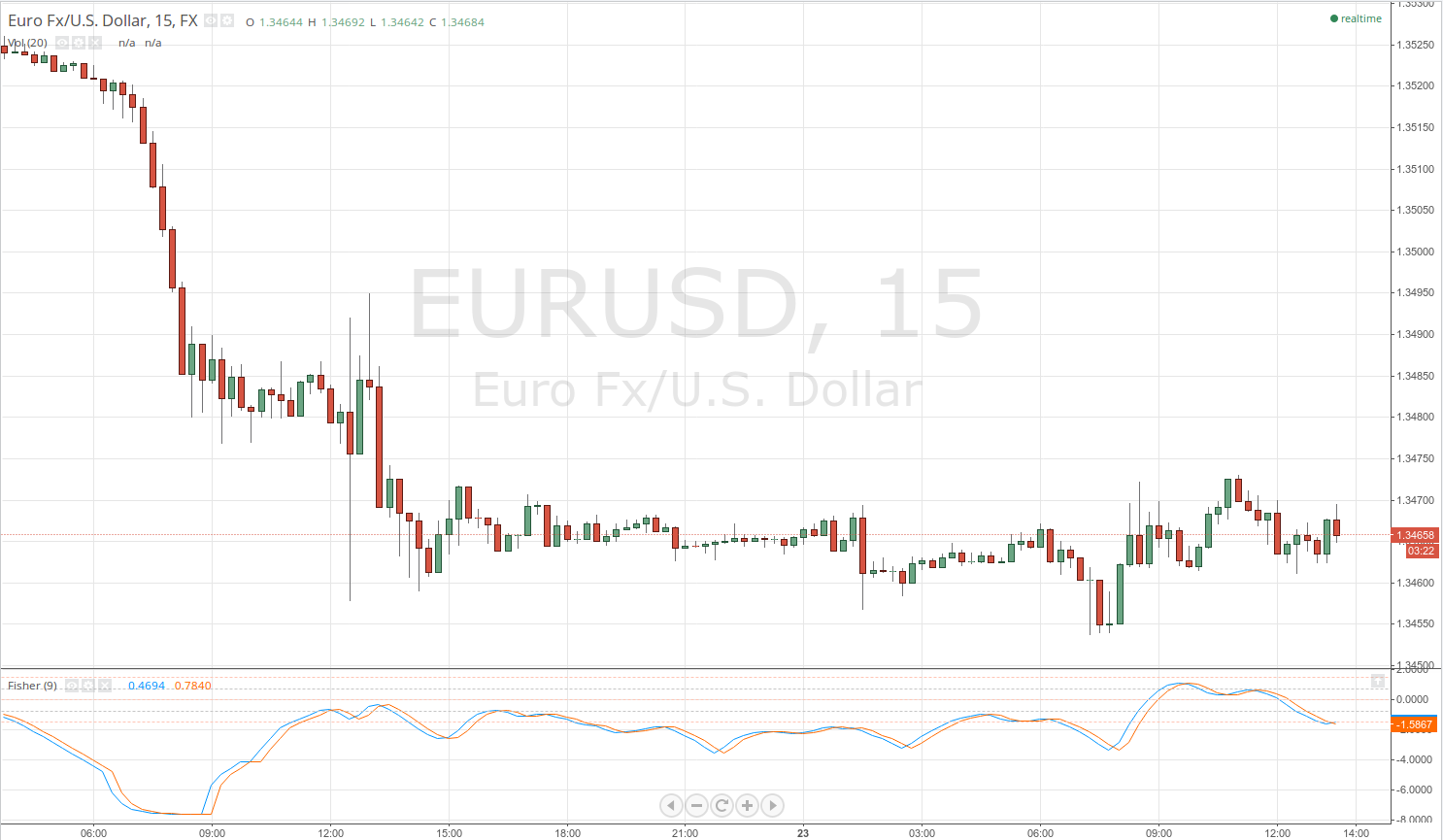# Ehlers Fisher Transform

## Ehlers Fisher Transform

### This lesson will cover the following

• Definition
• Interpretation

Developed by John Ehlers, the Fisher transform is a leading indicator designed to clearly spot major price reversals and visualize them with its distinct and sharp turning points which reflect spots where the rate of change is the biggest.

It is based on the assumption that prices do not have a normal probability density function (Gaussian PDF), although a common assumption is that they actually do. A Gaussian Probability Density Function is the bell-shaped curve of distribution where 68% of the samples fall within one standard deviation around the mean.

## Best Forex Brokers for United States

However, prices do not have a Gaussian PDF, which is where the Fisher transformation comes in. It changes the probability density function (PDF) of each waveform and the result has a Gaussian PDF.

This adds value to trading because when prices are normalized to fall between -1 and + 1 and are subjected to the Fisher transform, extreme price movements rarely occur, thus they can be much more easily defined. Turning points are sharp and distinct, and can be entered with a minor delay, thus improving the return on successful trades.

The indicator is visualized by two lines – the Fisher transform line and a signal line, whose crossovers generate entry signals much like the stochastic oscillators fast and slow lines. However, like many other indicators, especially the leading ones, it is not fool-proof and is prone to whipsaws which generate false signals. Thus, the Fisher transform is best used in a combination with other indicators to achieve better performance. An example is posted below.